# Newton's LawsPage 1

#### WATCH ALL SLIDES

Slide 1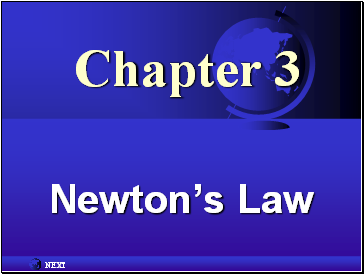Chapter 3

Newton’s Law

Slide 2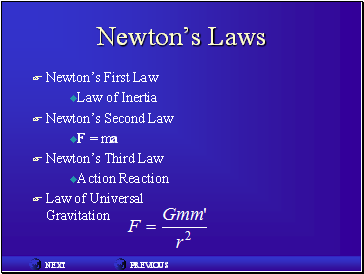## Newton’s Laws

Newton’s First Law

Law of Inertia

Newton’s Second Law

F = ma

Newton’s Third Law

Action Reaction

Law of Universal Gravitation

Slide 3## Mass

…is measured in kilograms.

…is the measure of the inertia of an object.

Inertia is the natural tendency of a body resist changes in motion.

Slide 4## Force

…the agency of change.

…changes the velocity.

…is a vector quantity.

.measured in Newton’s.

Slide 5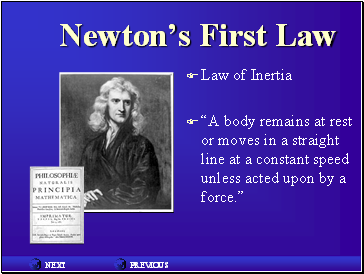## Newton’s First Law

Law of Inertia

“A body remains at rest or moves in a straight line at a constant speed unless acted upon by a force.”

Slide 6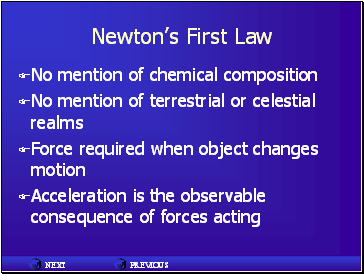Newton’s First Law

No mention of chemical composition

No mention of terrestrial or celestial realms

Force required when object changes motion

Acceleration is the observable consequence of forces acting

Slide 7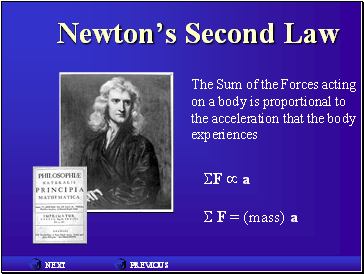## Newton’s Second Law

The Sum of the Forces acting on a body is proportional to the acceleration that the body experiences

F  a

S F = (mass) a

Slide 8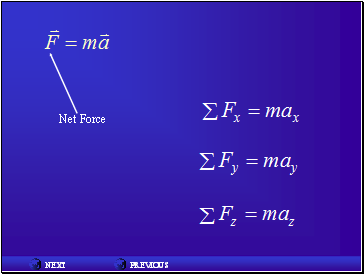Net Force

Slide 9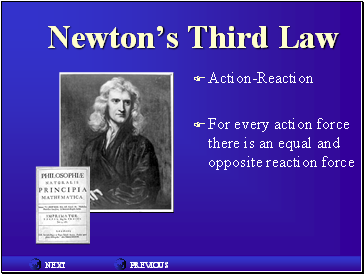## Newton’s Third Law

Action-Reaction

For every action force there is an equal and opposite reaction force

Slide 10## The Law of Gravity

Every mass exerts a force of attraction on every other mass.

The math…

G = 6.67  10-11 N·m2/kg2

Slide 11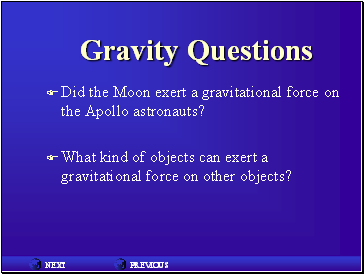## Gravity Questions

Did the Moon exert a gravitational force on the Apollo astronauts?

What kind of objects can exert a gravitational force on other objects?

Slide 12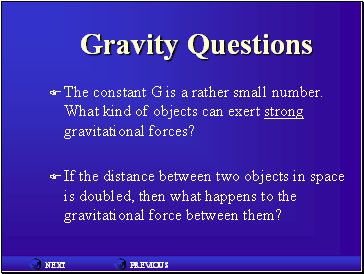Gravity Questions

The constant G is a rather small number. What kind of objects can exert strong gravitational forces?

If the distance between two objects in space is doubled, then what happens to the gravitational force between them?

Slide 13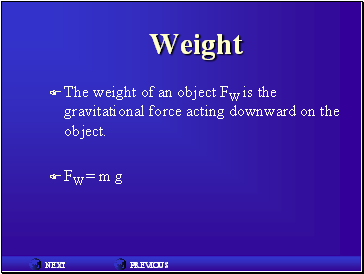Go to page:
1  2